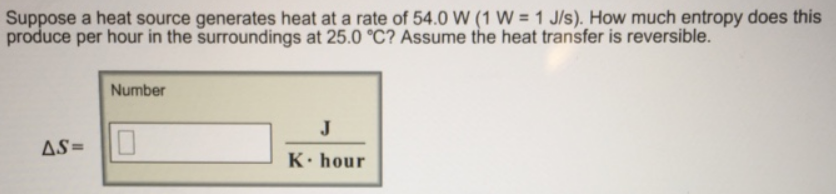Chemistry Practice Problems Entropy Practice Problems Solution: Suppose a heat source generates heat at a rate of ...

# Solution: Suppose a heat source generates heat at a rate of 54.0 W (1 W = 1 J/s). How much entropy does this produce per hour in the surroundings at 25.0 °C? Assume the heat transfer is reversible.

###### Problem

Suppose a heat source generates heat at a rate of 54.0 W (1 W = 1 J/s). How much entropy does this produce per hour in the surroundings at 25.0 °C? Assume the heat transfer is reversible.View Complete Written Solution

Entropy

Entropy

#### Q. Use the data from this table of thermodynamic properties to calculate the values of ΔS°rxn for each of the following reactions at 25° C. 2CO(g) + O2(g...

Solved • Tue Jun 26 2018 16:12:40 GMT-0400 (EDT)

Entropy

#### Q. Calculate ΔS° compositefunction (in J/mol • K) for the following reaction. 2HCl (g) + Pb (s) → PbCl2 (s) + H2 (g)

Solved • Mon Jun 25 2018 12:25:36 GMT-0400 (EDT)

Entropy

#### Q. Which of the following processes shows a decrease in entropy of the system? NaClO3 (s) → Na+ (aq) ClO3- (aq) CH3OH (l) → CO (g) + 2H 2 (g) H2O(l) → H2...

Solved • Mon Jun 04 2018 15:23:30 GMT-0400 (EDT)

Entropy

#### Q. Given the hypothetical reaction. 4 X(s) + 6Y(s) → 4Z (s) + 9A(g) Calculate Δ S for this reaction at 25°C, given the following absolute entropies: S°J/...

Solved • Wed May 30 2018 17:16:02 GMT-0400 (EDT)